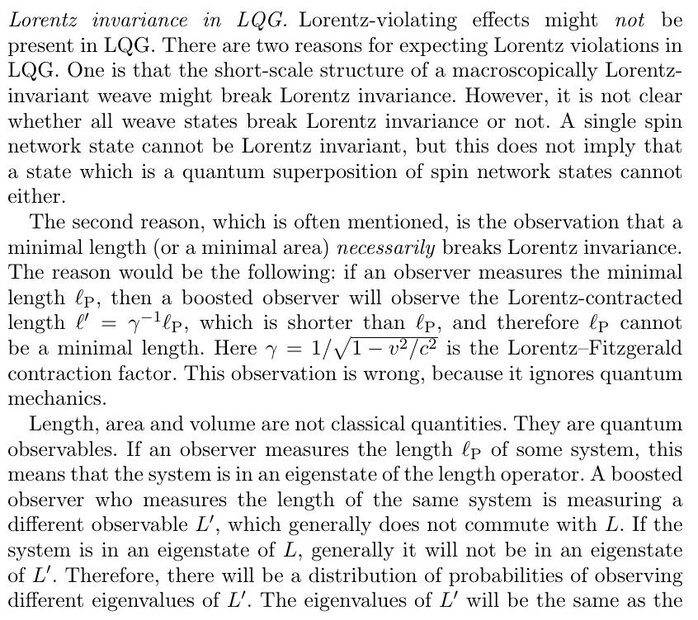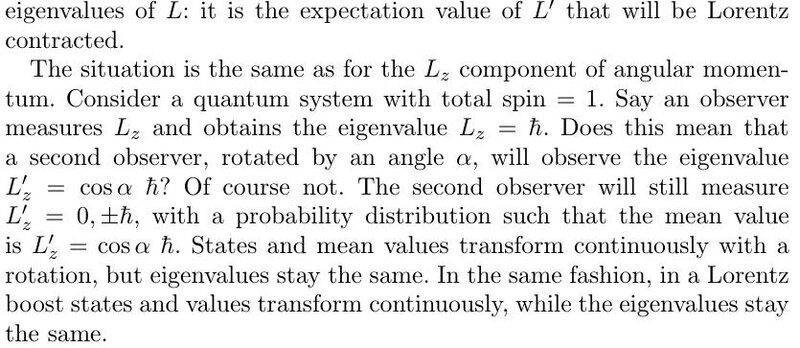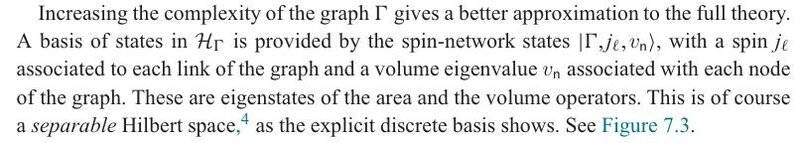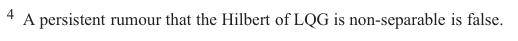# Rovelli on Quantum Gravity

• I
• Featured
I just went and looked at the five most recent papers on hep-th. All of them acknowledged support from some kind of grant, in countries as different as US, Japan, China, various EU, and Chile.

•ohwilleke and Demystifier
Summary:: One of the leading researchers in Loop Quantum Gravity discusses existing observations that should guide future quantum gravity research in a three page paper.

stop working on the anti-deSitter/conformal field theory relationship in gravity and cosmology work
I don't see that he's saying that. AdS/CFT never made any claim that our universe should be AdS. Their claim is that the duality defines a quantum gravity theory, which happens to live in AdS. Hopefully, gravity in our world works more-or-less similarly to how it works in AdS, at least as far as the high-energy stuff is concerned; so finding a full quantum gravity for AdS would be a great and very relevant achievement.

I understood Rovelli's remark to be referring to the "swampland" problem, that string theories with Calabi-Yau compactifications seem to predict a negative cosmological constant.

•Demystifier
Demystifier
Gold Member
Hopefully, gravity in our world works more-or-less similarly to how it works in AdS
Yes. AdS is somewhat like a harmonic oscillator; our world is not exactly a harmonic oscillator, but understanding of the harmonic oscillator helps a lot to understand the real world as well.

martinbn
Yes. AdS is somewhat like a harmonic oscillator; our world is not exactly a harmonic oscillator, but understanding of the harmonic oscillator helps a lot to understand the real world as well.
Is it like the harmonic oscillator or is it hoped to be?

Demystifier
Gold Member
Is it like the harmonic oscillator or is it hoped to be?
Good point, it's more like a hope.

So is Rovelli arguing against LQG now? As far as I'm concerned, it isn't locally Lorentz-invariant either, due to the inevitable singular excitations of geometry, at least at sufficiently small scales.

martinbn
So is Rovelli arguing against LQG now? As far as I'm concerned, it isn't locally Lorentz-invariant either, due to the inevitable singular excitations of geometry, at least at sufficiently small scales.
How so!?

How so!?
Local Lorentz-invariance means that spacetime is locally Minkowski, i.e. given any point in spacetime and any desired accuracy, there must be a small neighborhood around that point such that this neighborhood is isometric to a region of Minkowski spacetime, at least up to the specified accuracy. However, in all LQG models, geometry is defined by spin networks or spin foams. These are lattice-like structures embedded into the spacetime manifold. If you choose a point directly on a spin network, you will find its geometry to be excited. But if you pick any neighborhood of that point and any point within that neighborhood (other than the ones that lie on the lattice), the geometry around this other point will not be excited. There is a discontinuous jump. So there is no neighborhood around the points that lie on a spin network/foam that are isometric to a region of Minkowski spacetime. Minkowski spacetime is translation-invariant. You would have to be able to shift one point onto each other and still have the same geometry. But that's impossible due to the discontinuity.

I see a conceptual difference in violating a continous symmetry due to that it is in fact violated(and spacetime still is a justified continuum), or just because the continuum model may not be physically justified. Then it seems that, at before point where the symmetry is "violated", the set which is subject to the symmetry is no longer well defined. I think is may be more of a problem for the continuum model itself.

/Fredrik

martinbn
Local Lorentz-invariance means that spacetime is locally Minkowski, i.e. given any point in spacetime and any desired accuracy, there must be a small neighborhood around that point such that this neighborhood is isometric to a region of Minkowski spacetime, at least up to the specified accuracy.
This of course is not true. The neighborhood will be homeomerphic not isometric to a region in Minkowski.
However, in all LQG models, geometry is defined by spin networks or spin foams. These are lattice-like structures embedded into the spacetime manifold. If you choose a point directly on a spin network, you will find its geometry to be excited. But if you pick any neighborhood of that point and any point within that neighborhood (other than the ones that lie on the lattice), the geometry around this other point will not be excited. There is a discontinuous jump. So there is no neighborhood around the points that lie on a spin network/foam that are isometric to a region of Minkowski spacetime. Minkowski spacetime is translation-invariant. You would have to be able to shift one point onto each other and still have the same geometry. But that's impossible due to the discontinuity.

•weirdoguy
This of course is not true. The neighborhood will be homeomerphic not isometric to a region in Minkowski.
No, it must be isometric, i.e. not only topologically isomorphic, but the metric must be locally Minkowski. You need a homeomorphism which maps the local metric onto a Minkowski metric (up the the desired accuracy), hence an isometry.
You can check Thiemann's book for example. Any neighborhood of an at least trivalent vertex of a spin network has a finite volume, whereas any small enough neighborhood of a point that doesn't lie on the spin network, has zero volume. Hence, the whole volume is concentrated on the points that lie on the spin network.

Here's another way to look at it: Loop quantum gravity models are lattice models, just like lattice QCD. Sure, LQG gives to the freedom to choose the lattices as fine as you want to and you're not limited to regular lattices and can pick arbitrary graphs, but after you have made the choice, they remains lattices. Hence, some continuous symmetries such as local translation invariance are broken, just like in lattice QCD. The hope is that they are restored on macroscopic scales where the granularity of the lattice becomes irrelevant.

Last edited:
Demystifier
Gold Member
So is Rovelli arguing against LQG now? As far as I'm concerned, it isn't locally Lorentz-invariant either, due to the inevitable singular excitations of geometry, at least at sufficiently small scales.
Local Lorentz-invariance means that spacetime is locally Minkowski, i.e. given any point in spacetime and any desired accuracy, there must be a small neighborhood around that point such that this neighborhood is isometric to a region of Minkowski spacetime, at least up to the specified accuracy. However, in all LQG models, geometry is defined by spin networks or spin foams. These are lattice-like structures embedded into the spacetime manifold. If you choose a point directly on a spin network, you will find its geometry to be excited. But if you pick any neighborhood of that point and any point within that neighborhood (other than the ones that lie on the lattice), the geometry around this other point will not be excited. There is a discontinuous jump. So there is no neighborhood around the points that lie on a spin network/foam that are isometric to a region of Minkowski spacetime. Minkowski spacetime is translation-invariant. You would have to be able to shift one point onto each other and still have the same geometry. But that's impossible due to the discontinuity.•martinbn
It seems like he admits that Lorentz-violation effects might be present. In fact, I'm not arguing against his second argument, which I agree with. I'm arguing that "the short-scale structure of a macroscopically Lorentz-invariant weave might break Lorentz-invariance" as he puts it. He then argues that superpositions might help, but he doesn't provide a proof and it is easy to see that it isn't true: In the LQG Hilbert space, there is an uncountable number of orthogonal spin network states, but at most a countably infinite subset of them is allowed in a superposition. If the state is supposed to be Lorentz-invariant, it is necessary that each spin network in the superposition must be mapped onto another spin network that was already in the superposition). However, there is a continuum of Lorentz transformations and they generate a continuum of new spin network states when acted on a spin network state. Since only countable sums of spin network states are allowed in the LQG Hilbert space, not all of those transformed spin networks can be present in the sum, so there exist Lorentz transformations that don't leave the state invariant.

martinbn
No, it must be isometric, i.e. not only topologically isomorphic, but the metric must be locally Minkowski. You need a homeomorphism which maps the local metric onto a Minkowski metric (up the the desired accuracy), hence an isometry.
This is not the case. Otherwise the manifold will have zero curvature, and definitely not all have zero curvature. In fact "most" often it is not the case.

martinbn
It seems like he admits that Lorentz-violation effects might be present. In fact, I'm not arguing against his second argument, which I agree with. I'm arguing that "the short-scale structure of a macroscopically Lorentz-invariant weave might break Lorentz-invariance" as he puts it. He then argues that superpositions might help, but he doesn't provide a proof and it is easy to see that it isn't true: In the LQG Hilbert space, there is an uncountable number of orthogonal spin network states, but at most a countably infinite subset of them is allowed in a superposition. If the state is supposed to be Lorentz-invariant, it is necessary that each spin network in the superposition must be mapped onto another spin network that was already in the superposition). However, there is a continuum of Lorentz transformations and they generate a continuum of new spin network states when acted on a spin network state. Since only countable sums of spin network states are allowed in the LQG Hilbert space, not all of those transformed spin networks can be present in the sum, so there exist Lorentz transformations that don't leave the state invariant.
What happens in the case of QFT with a seperable Hilbert space? Are the states invariant? There are still a continuum of boosts.

This is not the case. Otherwise the manifold will have zero curvature, and definitely not all have zero curvature. In fact "most" often it is not the case.
Which is why I wrote "up to the desired accuracy." If you choose pick a desired ##\epsilon##, there must be neighborhood such that there exists a homeomorphism that is an isometry up to the given accuracy ##\epsilon##.
What happens in the case of QFT with a seperable Hilbert space? Are the states invariant? There are still a continuum of boosts.
The QFT Hilbert space is separable. All states can be expanded in a countable basis, so if you apply a boost to a sum of basis vectors, you can expand the resulting state in the same basis. That's no longer true in LQG. The set of spin network states forms a basis, but it is uncountable. A transformed spin network is orthogonal to the original spin network even if you change it only a tiny bit. There are no infinitesimal diffeomorphisms in LQG and the finite ones are discontinuous.

Demystifier
Gold Member
The set of spin network states forms a basis, but it is uncountable.
Rovelli and Vidotto in the book "Covariant Loop Quantum Gravity" say the opposite:•gentzen and weirdoguy
Rovelli and Vidotto in the book "Covariant Loop Quantum Gravity" say the opposite:
View attachment 293072View attachment 293073
Rovelli and Vidotto is about a different model than the one discussed in Rovelli or Thiemann. The model in Rovelli or Thiemann definitely has an uncountable basis. An uncountable basis is necessary if all diffeomorphisms are to be implemented as unitary transformations.

Demystifier
Gold Member
The model in Rovelli or Thiemann definitely has an uncountable basis.
Can you support this claim by a reference?

An uncountable basis is necessary if all diffeomorphisms are to be implemented as unitary transformations.
Why would diffeomorfisms would be implemented as unitary transformations in the first place? Shouldn't diffeomorphism equivalent states be counted as the same state in the Hilbert space?

Can you support this claim by a reference?
See Thiemann, p. 241: "We remark that the spin-network basis is not countable because the set of graphs in ##\sigma## is not countable, whence ##\mathcal H_0## is not separable."
Why would diffeomorfisms would be implemented as unitary transformations in the first place? Shouldn't diffeomorphism equivalent states be counted as the same state in the Hilbert space?
You first need to construct the kinematical Hilbert space, where the constraints are represented. The constraint algebra of GR contains the infinitesimal diffeomorphisms as subalgebra, so there must be a representation of the diffeomorphism group on the kinematical Hilbert space. In fact, in LQG, there is only a representation of the group and not the algebra, because LQG uses a discontinuous representation. No infinitesimal generators exist. After solving the diffeomorphism constraints, you end up on a diffeomorphism invariant Hilbert space, but it is still non-separable (see Thiemann as well).

Also check out Ashtekar, Lewandowski, "Background Independent Quantum Gravity: A Status Report":
"Note that there are continuous families of 4 or higher valent graphs which can not be mapped to one another by C n diffeomorphisms with n > 0. Consequently, states in Hdiff based on two of these graphs are mutually orthogonal. Thus, even though we have ‘factored out’ by a very large group Diff, the Hilbert space Hdiff is still non-separable."

•Demystifier
I'm not a dedicated fan of LQG anyway, but I noted this paper as well which seems far more recent than then Ashtekar/Lewandowski review from 2004? Any takes on this?

Separable Hilbert space in Loop Quantum Gravity
Winston Fairbairn, Carlo Rovelli, Oct 25, 2018

"...In the standard construction, the kinematical Hilbert space of the diffeomorphism-invariant states is nonseparable. This is a consequence of the fact that the knot-space of the equivalence classes of graphs under diffeomorphisms is noncountable. However, the continuous moduli labeling these classes do not appear to affect the physics of the theory. We investigate the possibility that these moduli could be only the consequence of a poor choice in the fine-tuning of the mathematical setting. We show that by simply choosing a minor extension of the functional class of the classical fields and coordinates, the moduli disappear, the knot classes become countable, and the kinematical Hilbert space of loop quantum gravity becomes separable."

-- https://arxiv.org/abs/gr-qc/0403047

/Fredrik

•Demystifier
I'm not a dedicated fan of LQG anyway, but I noted this paper as well which seems far more recent than then Ashtekar/Lewandowski review from 2004? Any takes on this?
Your paper is also from 2004.
Separable Hilbert space in Loop Quantum Gravity
Winston Fairbairn, Carlo Rovelli, Oct 25, 2018

"...In the standard construction, the kinematical Hilbert space of the diffeomorphism-invariant states is nonseparable. This is a consequence of the fact that the knot-space of the equivalence classes of graphs under diffeomorphisms is noncountable. However, the continuous moduli labeling these classes do not appear to affect the physics of the theory. We investigate the possibility that these moduli could be only the consequence of a poor choice in the fine-tuning of the mathematical setting. We show that by simply choosing a minor extension of the functional class of the classical fields and coordinates, the moduli disappear, the knot classes become countable, and the kinematical Hilbert space of loop quantum gravity becomes separable."
In this papers, they enlarge the ##Diff(\sigma)## symmetry subgroup of GR (the full group also includes the symmetries generated by the Hamiltonian constraint) to include more symmetries. If you require invariance under a larger symmetry group, the space of solutions may become smaller and even separable. However, there is no justification for the enlarged symmetry subgroup. ##Diff(\sigma)## arises as the symmetry subgroup of classical GR during the Dirac constraint analysis, but the extended group mentioned in that paper does not. Sure, if you give yourself the freedom to extend the symmetry group during quantization, you can shrink to Hilbert space and even make it zero-dimensional if you want to. But there is no justification for enlarging the symmetry subgroup.

Moreover, making the Hilbert space separable doesn't even help. Separability is only necessary for local Lorentz-invariance, but not sufficient. The general argument still holds, because it just so happens that the geometry in LQG is concentrated on singular objects. Being able to expand the transformed state in the same basis as the original state doesn't automatically lead to the equality of the two states, it's just a necessary condition for equality. So even if one were able to restrict the state space to a separable subspace of ##\mathcal H_{diff}##, one would then still need to prove local Lorentz-invariance.

To the best of my knowledge, the closest that LQG people have gotten to implementing Lorentz-invariance in a variant of LQG is to formulate a theory with ##SL(2,\mathbb C)## symmetry and implementing Lorentz-invariance at the vertices of a spin foam. However, it doesn't even make sense to apply Lorentz transformations to points that are not vertices, so the crucial feature, i.e. having a whole local neighborhood to resemble a region of Minkowski spacetime, has not been achieved.

•Fra and Demystifier
Demystifier
Gold Member
@Nullstein Generally I find very interesting that LQG is quite different from QFT. I vaguely remember that somewhere I saw a statement that when one applies the LQG method of quantization to a simple harmonic oscillator, one obtains a result not equivalent to harmonic oscillator quantized by standard techniques. Do you perhaps know a more precise statement and the relevant reference?

@Nullstein Generally I find very interesting that LQG is quite different from QFT. I vaguely remember that somewhere I saw a statement that when one applies the LQG method of quantization to a simple harmonic oscillator, one obtains a result not equivalent to harmonic oscillator quantized by standard techniques. Do you perhaps know a more precise statement and the relevant reference?
Yes, quantization works very differently in LQG. The position eigenstates ##\left|x\right>## are normalizable and orthogonal (##\left<x|x'\right>=\delta_{xx'}##) as compared to ordinary quantum mechanics, where you have ##\left<x|x'\right>=\delta(x-x')## (##\delta_{xx'}## is the Kronecker delta instead of the Dirac delta). While it is still possible to define the position operator (##\hat x\left|x\right>=x\left|x\right>##), it becomes impossible to define the momentum operator in such a way that the canonical commutation relations are obeyed. Instead, one quantizes the exponentiated momentum operators (##e^{i \epsilon p} \rightarrow \widehat{e^{i \epsilon p}}##) and they still acts as a translation operators: ##\widehat{e^{i \epsilon p}}\left|x\right> = \left|x-\epsilon\right>## However, in this Hilbert space, ##\left|x-\epsilon\right>## is not in any sense close to ##\left|x\right>##. Hence, it becomes impossible to calculate the derivative of ##\widehat{e^{i \epsilon p}}## at ##\epsilon=0## and so the infinitesimal generator ##\hat p## doesn't exist. So how are you going to define the Hamiltonian of the free particle or of the harmonic oscillator? The LQG people just define ##\hat p_{LQG} = \frac{1}{2i\epsilon}\left(\widehat{e^{i \epsilon p}}-\widehat{e^{-i \epsilon p}}\right)## for some sufficiently small, but non-zero ##\epsilon##, which thus becomes a free parameter of the theory. The Hamiltonian of the harmonic oscillator is then defined as ##\hat H = \frac{1}{2}\hat p_{LQG}^2 + \frac{1}{2}x^2## and naturally, you get deviations from the standard theory if you do this.

For a reference, you can check out Nicolai et al. "LQG: an outside view"

•Demystifier
Demystifier